# Quiz 2 - Flashcards

Set Details Share
created 3 years ago by SkyRose
6,451 views
updated 3 years ago by SkyRose
Subjects:
chemistry
Page to share:
Embed this setcancel
COPY
code changes based on your size selection
Size:
X

1

What group (G) and period (P) does the element molybdenum (Mo) belong to?

A) G = 5, P = 6

B) G = 6, P = 6

C) G = 6, P = 4

D) G = 6, P = 5

E) G = 5, P = 5

D) G = 6, P = 5

2

Which of the following elements would be chemically similar to calcium (Ca)?

A) K

B) Sr

C) Sc

D) SE) Ga

B) Sr

3

Which of the following is NOT a metalloid?

A) Pb

B) B

C) Ge

D) Sb

E) Te

A) Pb

4

Which of the following elements is NOT a metal?

A) arsenic

B) indium

C) iridium

D) lithium

E) lutetium

A) arsenic

5

Which of the following is a main group element?

A) Yttrium

B) Osmium

C) Holmium

D) Californium

E) Bismuth

E) Bismuth

6

Which of the following is an alkaline earth metal?

A) Rubidium

B) Beryllium

C) Iridium

D) Americium

E) Phosphorous

B) Beryllium

7

Which periodic trend quantifies the amount of energy required to remove an electron from a neutral, gaseous atom?

B) Ionization Energy

D) Electron Affinity

E) Electronegativity

B) Ionization Energy

8

Which of the following transition metals would be expected to have the smallest atomic radius?

A) Yttrium (Y)

B) Zirconium (Zr)

C) Niobium (Nb)

D) Technetium (Tc)

E) Ruthenium (Ru)

E) Ruthenium (Ru)

9

Rank the following atoms in order of increasing first ionization energies (i.e., lowest to highest): Rb, F, Mg, B, N.

A) F < N < B < Mg < Rb

B) Rb < Mg < F < N < B

C) Mg < Rb < F < N < B

D) Rb < Mg < B < N < F

E) Rb > B > N > F > Mg

D) Rb < Mg < B < N < F

10

Rank the following atoms in order of increasing size (i.e., smallest to largest): Li, Al, Be, Ba, O.

A) Li < Al < Be < Ba < O

B) Ba < Al < Li < Be < OC) Li < Be < O < Al < Ba

D) O < Be < Li < Al < Ba

E) O < Li < Be < Ba < Al

D) O < Be < Li < Al < Ba

11

Predict the chemical formula for the ionic compound formed by Au³⁺ and HSO₃⁻

Au(HSO₃)₃

12

Predict the chemical formula for the ionic compound formed by Cu²⁺ and P³⁻

Cu₃P₂

13

Predict the chemical formula for the ionic compound formed by the elements Ag and O

Ag₂O

14

Predict the chemical formula for the ionic compound formed by the elements Ba and S

BaS

15

What is the formula of a compound containing Al³⁺ and S²⁻ ions?

A) Al₂S₃

B) Al₂S

C) AlS₃

D) Al₃S₂

A) Al₂S₃

16

Classify the following compounds as ionic or covalent: BaO, Fe₂O₃, ZnO.

A) Covalent, covalent, covalent.

B) Ionic, ionic, covalent.

C) Ionic, covalent, covalent.

D) Ionic, Ionic, Ionic.

D) Ionic, Ionic, Ionic.

17

Which of the following compounds exhibits both ionic and covalent bonding?

A) Cl₂O₇

B) KCl

C) KNO₃

D) N₂O₄

C) KNO₃

18

Which of the following is best classified as a covalent compound?A) Sr₃N₂

B) N₂O₅

C) Li₃N

D) AlN

B) N₂O₅

19

Which of the following compounds does NOT exhibit covalent bonding?

A) CO₂

B) COC) CoCl₂

D) HCOOH

E) Na₂CO₃

C) CoCl₂

20

Which of the following is a molecular compound?

A) P₄

B) BaBr₂

C) N₂O

D) CaO

E) Ag

C) N₂O

21

Sodium bicarbonate (baking soda, NaHCO₃) is used to deodorize refrigerators. What kind of bonding does this compound exhibit?

A) Ionic only

B) Covalent only

C) Both ionic and covalent

D) Metallic only

C) Both ionic and covalent

22

The compound NaBr is:

A) Ionic

B) Polar covalent

C) Nonpolar covalent

D) Metallic

E) Not enough info

A) Ionic

23

Which of the following is an example of an ionic bond?

A) K - F

B) C - H

C) Kr - H

D) C - F

A) K - F

24

What is the correct IUPAC name for Ag₂S?

silver sulfide

25

What is the correct IUPAC name for AlN?

aluminum nitride

26

What is the correct IUPAC name for BaS?

barium sulfide

27

What is the correct IUPAC name for K₂O

potassium oxide

28

What is the correct IUPAC name for MgF₂?

magnesium fluoride

29

What is the correct IUPAC name for Au₂O₃?

gold(III) oxide

30

What is the correct IUPAC name for CrCl₂?

chromium(II)chloride

31

What is the correct IUPAC name for CrCl₂?

chromium(II) chloride

32

What is the correct IUPAC name for FeCl₃?

iron(III) chloride

33

What is the correct IUPAC name for MnF₃?

manganese(III) fluoride

34

What is the correct IUPAC name for SnS₂?

tin(IV)sulfide

35

What is the correct IUPAC name for AlPO₄?

aluminum phosphate

36

What is the correct IUPAC name for Ca(NO₃)₂?

calcium nitrate

37

What is the correct IUPAC name for Li₃PO₄?

lithium phosphate

38

What is the correct IUPAC name for Na₂SO₃?

sodium sulfite

39

What is the correct IUPAC name for Co₂(SO₄)₃?

cobalt(III) sulfate

40

What is the correct IUPAC name for Fe(HSO₄)₃?

iron(III) hydrogen sulfate

41

What is the correct IUPAC name for SnSO₄?

tin(II) sulfate

42

What is the correct IUPAC name for CuClO₃?

copper(I) chlorate

43

What is the correct IUPAC name for Cr₂(CO₃)₃?

chromium(III) carbonate

44

What is the correct IUPAC name for LiOH?

lithium hydroxide

45

What is the correct IUPAC name for IF₅?

iodine pentafluoride

46

What is the correct IUPAC name for PBr₃?

phosphorus tribromide

47

What is the correct IUPAC name for SCl₂?

sulfur dichloride

48

What is the correct IUPAC name for SiCl₄?

silicon tetrachloride

49

What is the correct IUPAC name for S₂F₈?

disulfur octafluoride

50

Write the chemical formula for the chlorite ion

ClO₂⁻

51

Write the chemical formula for the nitrate ionNO³⁻

NO₃⁻

52

Write the chemical formula for ammonium hydroxide

NH₄OH

53

Write the chemical formula for calcium chloride

CaCl₂

54

Write the chemical formula for chromium(III) phosphate

CrPO₄

55

Write the chemical formula for cobalt(II) hydrogen carbonate

Co(HCO₃)₂

56

Write the chemical formula for copper(I) hydroxide

CuOH

57

Write the chemical formula for iron(III) oxideFe₂O₃

Fe₂O₃

58

Write the chemical formula for lead(II) nitrate

Pb(NO₃)₂

59

Write the chemical formula for potassium hydrogen carbonate

KHCO₃

60

Write the chemical formula for silver chloride

AgCl

61

Write the chemical formula for zinc nitrate

Zn(NO₃)₂

62

Write the chemical formula for carbon disulfide

CS₂

63

Write the chemical formula for disulfur decafluoride

S₂F₁₀

64

Write the chemical formula for phosphorus trihydride

PH₃

65

Write the chemical formula for sulfur dioxide

SO₂

66

Determine the correct formula for chromium(II) nitrite.

A) CrNO₂

B) Cr₂NO₂

C) Cr(NO₂)₂

D) Cr₃(NO₂)₂

C) Cr(NO₂)₂

67

Determine the correct formula for manganese(II) fluoride.

A) MnF

B) MnF₂

C) MnF₃

D) Mn₂F

B) MnF₂

68

Determine the correct formula for titanium(IV) chloride.

A) TiCl₂

B) Ti₄Cl

C) Ti₂Cl₄

D) TiCl₄

D) TiCl₄

69

Which has the correct name-formula combination?

A) Iron(II) nitride - FeN

B) Nickel(II) oxide - NiO₂

C) Titanium(IV) phosphide - TiP

D) Manganese(II) sulfide - MnS

D) Manganese(II) sulfide - MnS

70

Which has the incorrect name-formula combination?

A) Iron(III) fluoride - FeF₃

B) Cobalt(II) oxide - CoO

C) Copper(I) sulfide - CuS

D) Chromium(II) bromide - CrBr₂

C) Copper(I) sulfide - CuS

71

Which has the incorrect name-formula combination?

A) Chromium(II) sulfate - CrSO₄

B) Cobalt(III) nitrate - Co(NO₃)₂

C) Nickel(II) nitrite - Ni(NO₂)₂

D) Manganese(III) sulfite - Mn₂(SO₃)₃

B) Cobalt(III) nitrate - Co(NO₃)₂

72

Which of the following is the correct Lewis symbol for a neutral atom of Mg?

A) see problem image

B) see problem image

C) see problem image

D) see problem image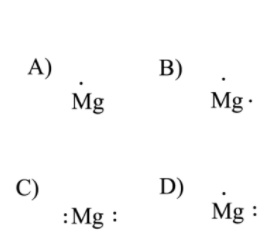B) see problem image

73

Which of the following is the correct Lewis symbol for the calcium ion?

A) see problem image

B) see problem image

C) see problem image

D) see problem image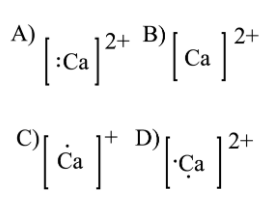B) see problem image

74

Which of the following is the correct Lewis symbol for the nitride ion?

A) see problem image

B) see problem image

C) see problem image

D) see problem image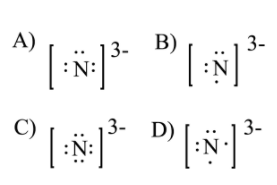C) see problem image

75

Which of the following is the correct Lewis symbol for the nitride ion?

A) see problem image

B) see problem image

C) see problem image

D) see problem image

C) see problem image

76

Identify which of the following molecules has a double bond.

A) O₂

B) H₂

C) Br₂

D) HF

A) O₂

77

Draw the Lewis structure of HF. Please place the elements in the order that they are written.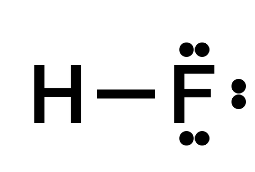78

Draw the Lewis structure of methane (CH₄).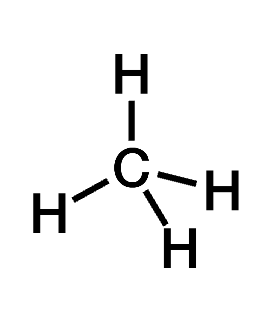79

Draw the Lewis structure of NF₃.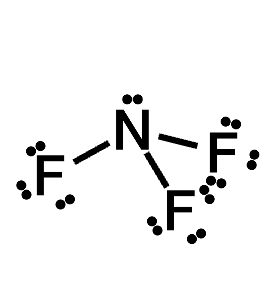80

Determine the number of valence electrons in CH₂Cl₂ and then draw the corresponding Lewis structure.

A) 23

B) 20

C) 16

D) 22

E) 24B) 20

81

Determine the number of valence electrons in CH₂Cl₂ and then draw the corresponding Lewis structure.

A) 23

B) 20

C) 16

D) 22

E) 24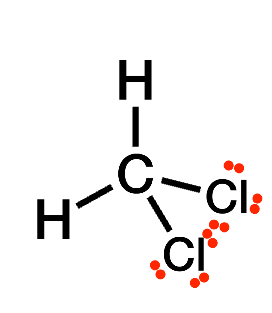B) 20

82

Determine the number of valence electrons in SiF₄ and then draw the corresponding Lewis structure.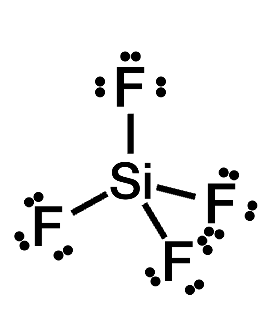C) 32

83

The Lewis structure for OCl₂ shown below is incorrect. Starting from this structure, complete the correct structure.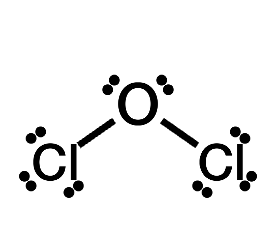84

Determine the number of valence electrons in NH₂OH and then draw the corresponding Lewis structure.

A) 16

B) 14

C) 13

D) 10

E) 15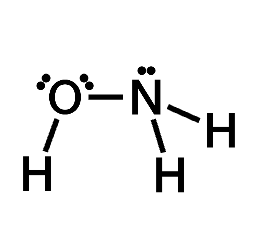B) 14

85

Determine the number of valence electrons in sulfuric acid (H₂SO₄) and then draw the corresponding Lewis structure (by following the octet rule on all atoms).

A) 32

B) 28

C) 36

D) 33

E) 30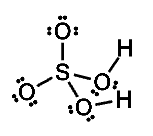A) 32

86

Which of the following is the strongest type of bond?

A) a single bond

B) a double bond

C) a triple bond

C) a triple bond

87

Which of the following has the shortest bond length?

A) a single bond

B) a double bond

C) a triple bond

C) a triple bond

88

Which one of the following is the most electronegative atom?

A) C

B) N

C) O

D) F

D) F

89

Based on their positions in the periodic table, which of the following bonds is the most polar?

A) C - O

B) C - N

C) C - B

D) C - F

D) C - F

90

Which one of the following statements is FALSE?

A) In a polar covalent bond, the electrons in the bond will be closer to the more electronegative atom

B) In a nonpolar covalent bond, the electrons in the bond are equally balanced between the two atoms

C) In a bond between boron and hydrogen, the electrons will be unequally shared

D) A bond between nitrogen and oxygen will be polar

E) In a bond between magnesium and sulfur, the electrons will be shared and closer to the sulfur

E) In a bond between magnesium and sulfur, the electrons will be shared and closer to the sulfur

91

Draw the Lewis structure of CBr₄ and then determine its electron domain and molecular geometries.Click to edit molecule

A) trigonal / planar

B) linear / trigonal pyramidal

C) tetrahedral / tetrahedral

D) planar / trigonal

E) planar / tetrahedral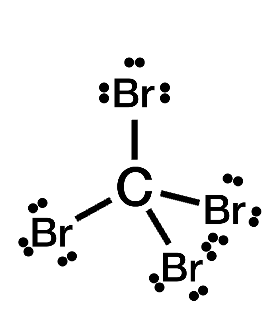C) tetrahedral / tetrahedral

92

Draw the Lewis structure of HCN and then determine its electron domain and molecular geometries.Click to edit molecule

A) tetrahedral / planar

B) trigonal planar / tetrahedral

C) tetrahedral / bent (109.5º)

D) linear / linear

E) bent (120º) / bent (109.5º)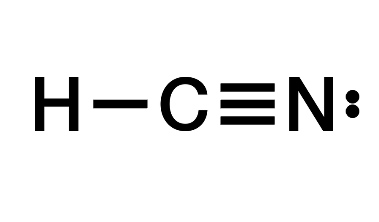D) linear / linear

93

Draw the Lewis structure of SF₄ and then determine its electron domain and molecular geometries.Click to edit molecule

A) trigonal planar / tetrahedral

B) trigonal / trigonal planar

C) pyramidal / pyramidal

D) trigonal bipyramidal / see-saw

E) trigonal / bent (109.5º)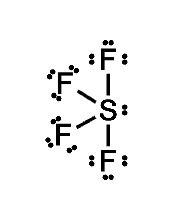D) trigonal bipyramidal / see-saw

94

Draw the Lewis structure of OF₂ and then determine the ideal bonding angle(s) of the central atom.Click to edit molecule

A) 135º

B) 45º

C) 120º

D) 60º

E) 109.5º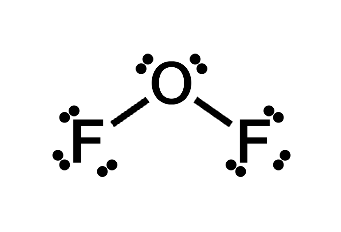E) 109.5º

95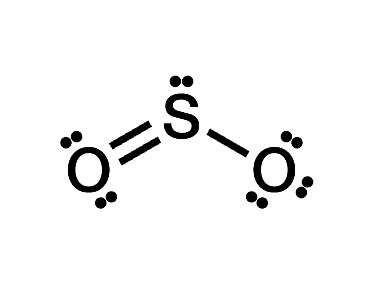Draw the Lewis structure of SO₂ (by following the octet rule on all atoms) and then determine the ideal bonding angle(s) of the central atom.Click to edit molecule

A) 109.5º

B) 180º

C) 120º

D) 60º

E) 30º

C) 120º

96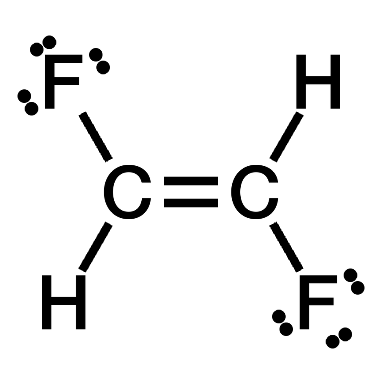A Lewis structure for a different isomer C₂H₂F₂ is shown below. Based on this structure, determine if the molecule is polar or nonpolar.

A) Nonpolar

B) Polar

A) Nonpolar

97

A Lewis structure for the molecule phenol (C₆H₅OH) is shown below. Based on this structure, determine if the molecule is polar or nonpolar.

A) Nonpolar

B) Polar

B) Polar

98

Draw the Lewis structure of CH₃F and then determine if the molecule is polar or nonpolar.

B) Polar

99

A Lewis structure for one isomer C₂H₂F₂ is shown below. Based on this structure, determine if the molecule is polar or nonpolar.

A) Nonpolar

B) Polar

B) Polar

100

Draw the Lewis structure of carbon monoxide (CO) and then determine if the molecule is polar or nonpolar. Please place the elements in the order that they are written.

A) Nonpolar

B) Polar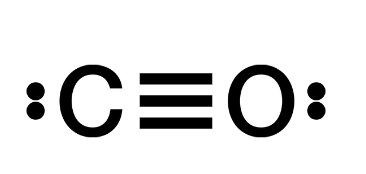B) Polar

101

Which one of the following best describes the difference between molar mass and formula weight?

A) Formula weights are measured in grams per mole, while molar masses are measured in atomic mass units.

B) A formula weight is the mass of one molecule or formula unit while a molar mass is the mass of one mole of molecules or formula units.

C) Molar masses only describe the mass of ionic compounds, while formula weights can describe any compound.

D) There is no difference between molar masses and formula weights.+

B) A formula weight is the mass of one molecule or formula unit while a molar mass is the mass of one mole of molecules or formula units.

102

Determine the formula weight of Ca(NO₃)₂

164.10 amu

103

Determine the molar mass of Fe(NO₃)₂.

179.85 g/mol

104

Which of the following has the greatest molar mass?

A) CaF₂

B) MgBr₂

C) CsF

D) RbCl

B) MgBr₂

105

A hydrocarbon is referred to as __________ if all the bonds in the molecule are single bonds.

A) aromatic

B) saturated

C) unsaturated

D) planar

E) octahedral

B) saturated